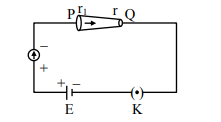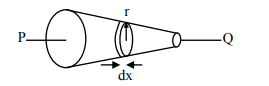# In the given figure, a battery of emf E is connected across a conductor PQ of length l`
Question:

In the given figure, a battery of emf $E$ is connected across a conductor PQ of length $l '$ and different area of cross-sections having radii $r_{1}$ and $r_{2}\left(r_{2}Choose the correct option as one moves from P to Q : 1. Drift velocity of electron increases. 2. Electric field decreases. 3. Electron current decreases 4. All of these Correct Option: 1 Solution:Current is constant in conductor i = constant Resistance of element$\mathrm{dR}=\frac{\rho \mathrm{dx}}{\pi \mathrm{r}^{2}}\mathrm{dV}=\mathrm{idR}=\frac{i \rho \mathrm{dx}}{\pi \mathrm{r}^{2}}\mathrm{E}=\frac{\mathrm{dV}}{\mathrm{dx}}=\frac{\mathrm{i \rho}}{\pi \mathrm{r}^{2}}\& V_{d}=\frac{e E \tau}{m}\therefore V_{d \propto} E\rightarrow \quad \mathrm{E} \propto \frac{1}{\mathrm{r}^{2}}$if$\mathrm{r}$decreases,$\mathrm{E}$will increase$\therefore \mathrm{V}_{\mathrm{d}}\$ will increase